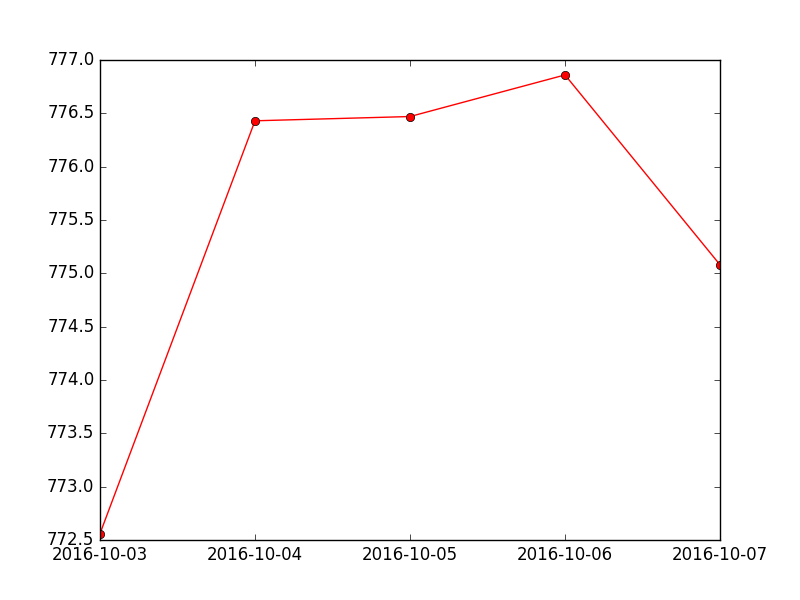﻿ Matplotlib Basic: Create multiple types of charts on a single set of axes - w3resource# Matplotlib Basic: Create multiple types of charts on a single set of axes

## Matplotlib Basic: Exercise-12 with Solution

Write a Python program to create multiple types of charts (a simple curve and plot some quantities) on a single set of axes.

Sample Solution:

Python Code:

``````import datetime as DT
from matplotlib import pyplot as plt
from matplotlib.dates import date2num

data = [(DT.datetime.strptime('2016-10-03', "%Y-%m-%d"), 772.559998),
(DT.datetime.strptime('2016-10-04', "%Y-%m-%d"), 776.429993),
(DT.datetime.strptime('2016-10-05', "%Y-%m-%d"), 776.469971),
(DT.datetime.strptime('2016-10-06', "%Y-%m-%d"), 776.859985),
(DT.datetime.strptime('2016-10-07', "%Y-%m-%d"), 775.080017 )]

x = [date2num(date) for (date, value) in data]
y = [value for (date, value) in data]

fig = plt.figure()

# Plot the data as a red line with round markers
graph.plot(x,y,'r-o')

# Set the xtick locations
graph.set_xticks(x)

# Set the xtick labels
graph.set_xticklabels(
[date.strftime("%Y-%m-%d") for (date, value) in data]
)
plt.show()
```
```

Sample Output:Python Code Editor: